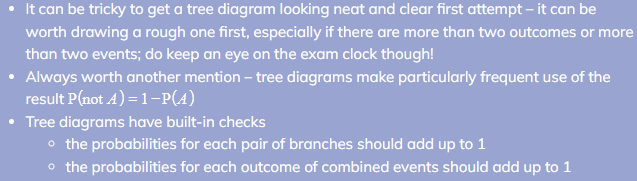# AQA A Level Maths: Statistics复习笔记3.2.3 Further Tree Diagrams

### Further Tree Diagrams

#### What do you mean by further tree diagrams?

• The tree diagrams used here are no more complicated than those in the first Tree Diagrams revision note, however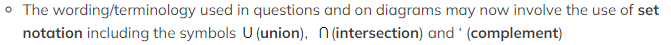• e.g.  P(A') would be used for “P(not A)”
• Conditional probability questions can be solved using tree diagrams

#### How do I solve conditional probability problems using tree diagrams?

•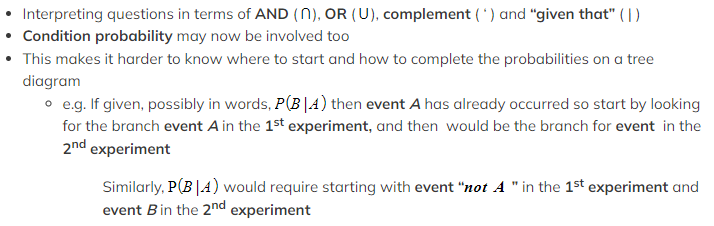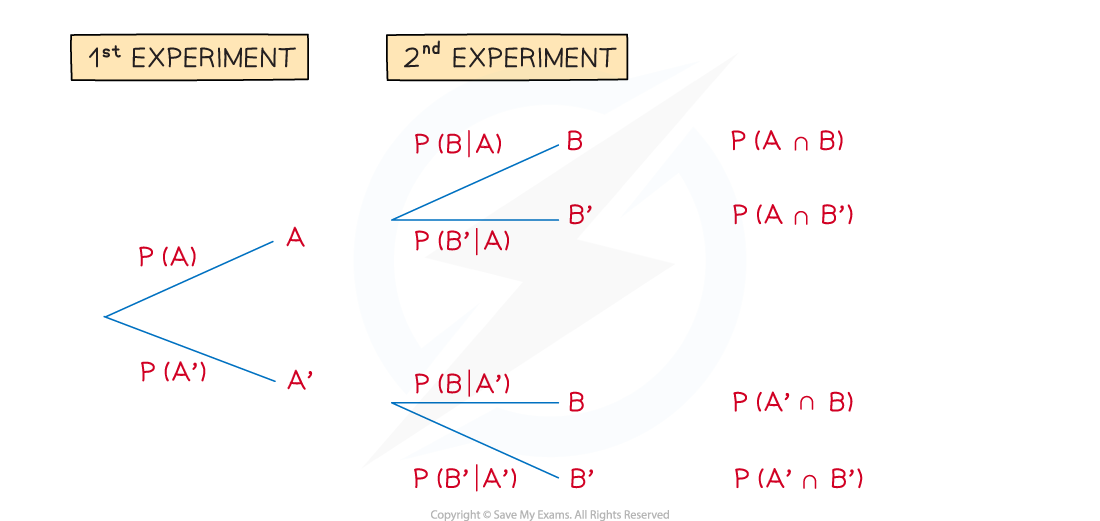####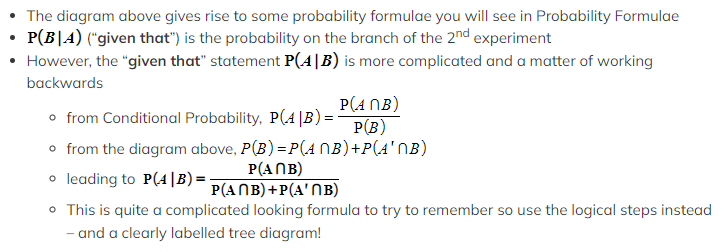Worked Example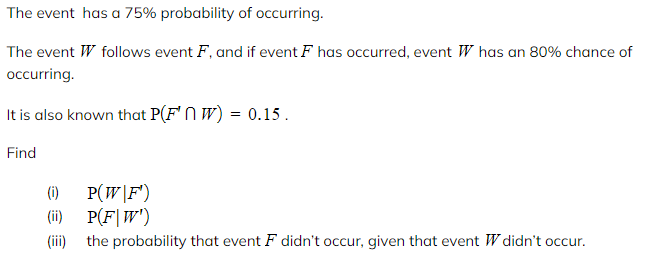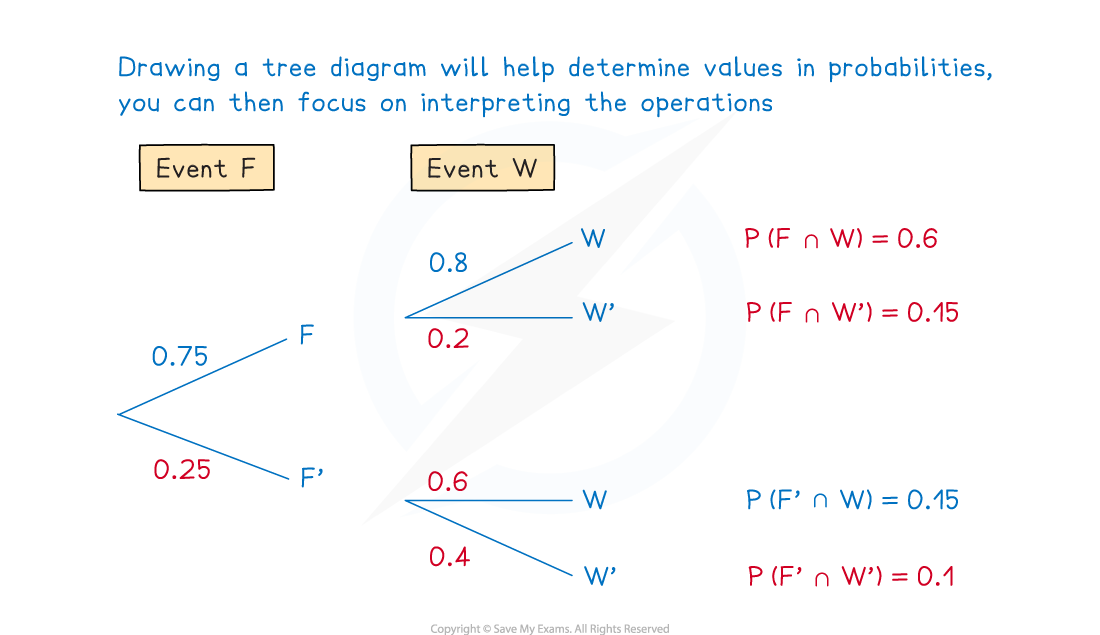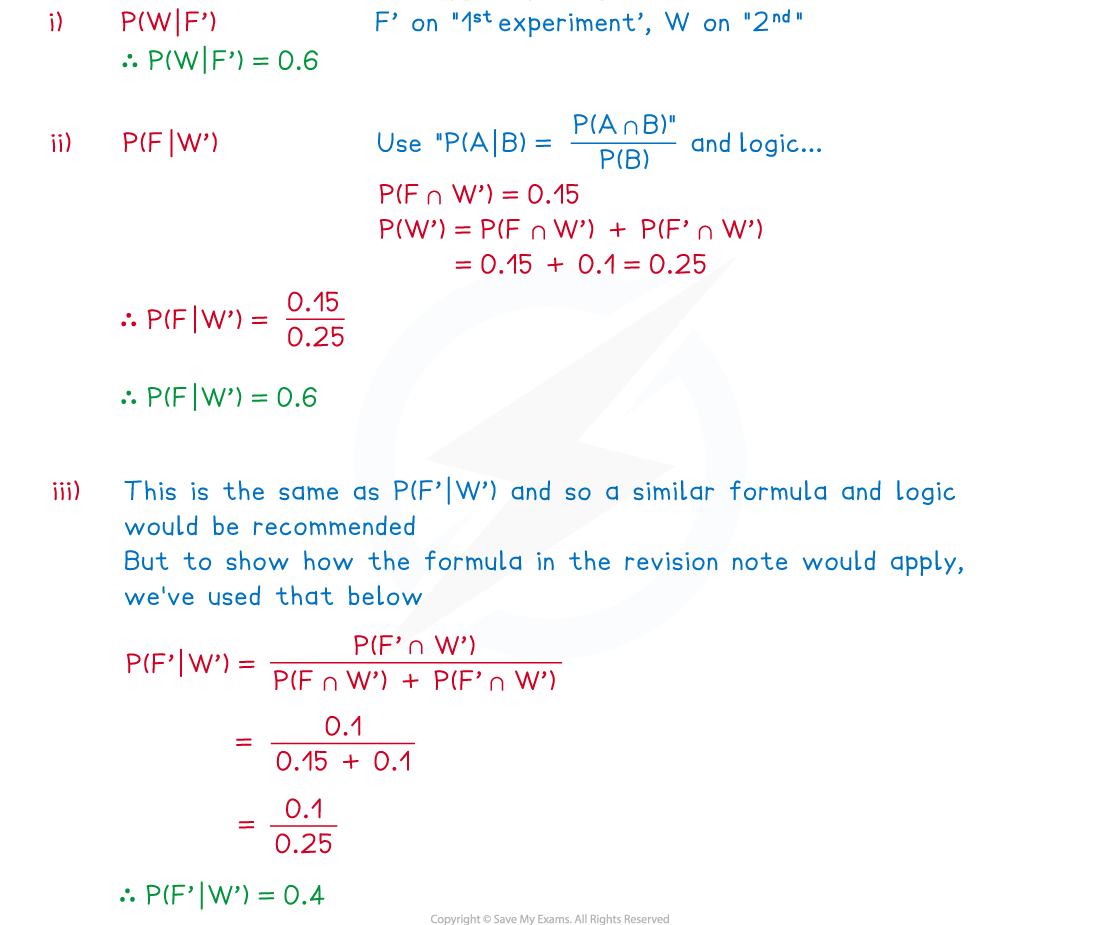#### Exam Tip